Real-time Analysis

In real-time analysis, FFT operation for sampled data is performed continuously without intervals between windows.

In usual FFT analysis, when sampling for the analysis data length (1024 or 2048 points) of signal has been performed, FFT operation for the data is performed. The next data is sampled during FFT operation and, when FFT operation for that data is completed, FFT operation for the next data starts. If the duration of FFT operation (including the time necessary for display) is shorter than the sampling time, real-time analysis can be performed. However, if the sampling time is shorter than the duration of FFT operation, a new signal for one ore more frames is sampled during operation resulting in missing signal. If the sampling time is longer than the duration of FFT operation, part of the window can be overlapped with the previous window (overlap processing).

Rotational Order Ratio Analysis

With the rotational order ratio analysis, signal sampling is performed using the pulse of the pulse generator attached to the body of rotation as an external sampling clock for the purpose of frequency analysis of vibration and noise of rotating machinery.

With frequency analysis, 1 Hz is a component which completes one cycle in one second. With rotational order ratio analysis, on the other hand, the rotational 1st order is a component which completes one cycle for each rotation of the reference body of rotation.
The rotational 2nd order is a component which completes two cycles for each rotation of the body of rotation, which is twice the case of the rotational 1st order. Thus, in order to perform analysis based on the variation for each rotation, sampling must be performed in synchronization with the revolution speed.  If only the internal sampling clock is used, the number of samples per rotation changes with the variation of the rotational speed. However, if a clock synchronizing with the rotation pulse is used as a sampling clock, the number of samples per rotation becomes constant.

For example, suppose a body of rotation with a rotational speed of 600 r/min. The rotational 1st order is (600 r/min)/60 = 10 Hz and the rotational 2nd order 20 Hz.  If the revolution speed reaches 700 r/min, the rotational 1st order increases to 11.7 Hz and the rotational 2nd order to 23.3 Hz.   Thus, the frequency changes with the variation of the rotational speed, However, normalizing the frequency as an order makes it easier to focus a certain component without being affected by the variation of the rotational speed.

Rotational Tracking Analysis

The rotational tracking analysis is an application of the rotational order ratio analysis.

The rotational tracking analysis is an analysis method which is used to determine which component of the rotating machine resonates or what time (what order) component of the rotational speed resonates, with respect to a certain rotational speed, by tracing the level variation of a certain order component as a horizontal-axis parameter.

Root Mean Square (RMS)

The root mean square denotes the mean of squares of the signal, which is calculated by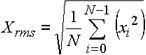With a sine wave, the RMS value is 1/√2 times the peak value. The data of each line of the power spectrum equals to the RMS value of the signal in the relevant frequency band.

RMS value

Take an electric heater as an example. When DC voltage is applied to the electric heater, it generates heat. Since the nichrome wire of the electric heater (or ceramic with recent ceramic heaters) is a resistive element (resistor), the circuit is as shown in Fig. 1.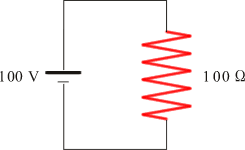Figure 1

Suppose the energy (heat) generated when the voltage is 100 V and the resistance is 100 ohms. From Ohm's law,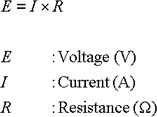The current flowing in the resistor is I=E/R=100/100=1(A), and the power W is W=E x I=100x1=100(W). Since the calculation of current is troublesome, the following expression is generally used from I=E/R: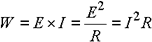When 100 V is applied to a 100-ohm electric heater, heat for 100 W is generated. In the Kanto area, the 100 V rms domestic power voltage is supplied, not as DC voltage but as 50 Hz AC voltage (sine wave). When a 100-ohm electric heater is connected to the 100 V power outlet, what amount of heat (in watts) is generated? The answer is 100 W, i.e., the RMS value is the equivalent DC voltage that performs the same work. So when the same electric heater is connected to a 200-V power outlet, what amount of heat (in watts) is generated? (Actually, the heater will be damaged.)
Since 200 VAC has the same power as 200 VDC, the power is 2002/100=400W, not 200W. When the voltage is doubled, the current is also doubled and the power is quadrupled (22). What is the RMS value for the following case in Fig. 2?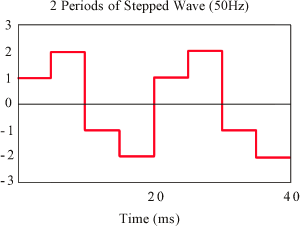Figure 2

The simple mean of the absolute value is 1.5 V.  Let us consider the power actually generated.   Since calculation is troublesome with a resistance of 100Ω, a resistance of 1Ω is assumed.   The power generated with 1V and 2V is given by the following expressions.  Even if the resistance (1Ω) is omitted, the result remains unchanged.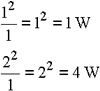In this case, the square of the voltage becomes the power generated at the resistor.  If the voltage or current is multiplied by itself, you may recognize the expression as the power. (In the case of 1Ω, the voltage and current are equal and therefore the square of the current also becomes the power from expression (2).)

The mean power is 2.5W because the 1W section is 1/2 and the 4W section is 1/2. as for mean power, the section of 1W is set to 2.5W.  That is, this waveform has the same power as the DC voltage that generates 2.5W power in the 1Ω resistor.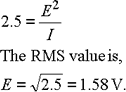The RMS value is the square root of the mean of the power.  In other words, it is the square root of the mean of the square value.  This is referred to as "root mean square." If you want to emphasize that the value is the RMS value, it is described as "rms."

Since the RMS value of a sine wave equals 1/√2=0.707 times the peak value, the waveform of the domestic 100 V power has a peak value of 141V which equals √2 times the RMS value. (The mean is 2/π=0.637.)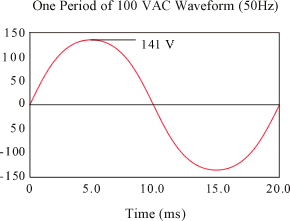Figure 3

Let us connect the 100-ohm electric heater to this power. The maximum power is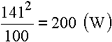Since it is apparent that the minimum power is 0 W, you can easily imagine that the power swings between 0 and 200W.   This phenomenon is shown in Fig. 4. It is apparent that the mean at this time is 100W.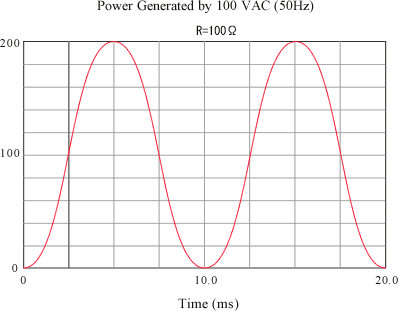Figure 4